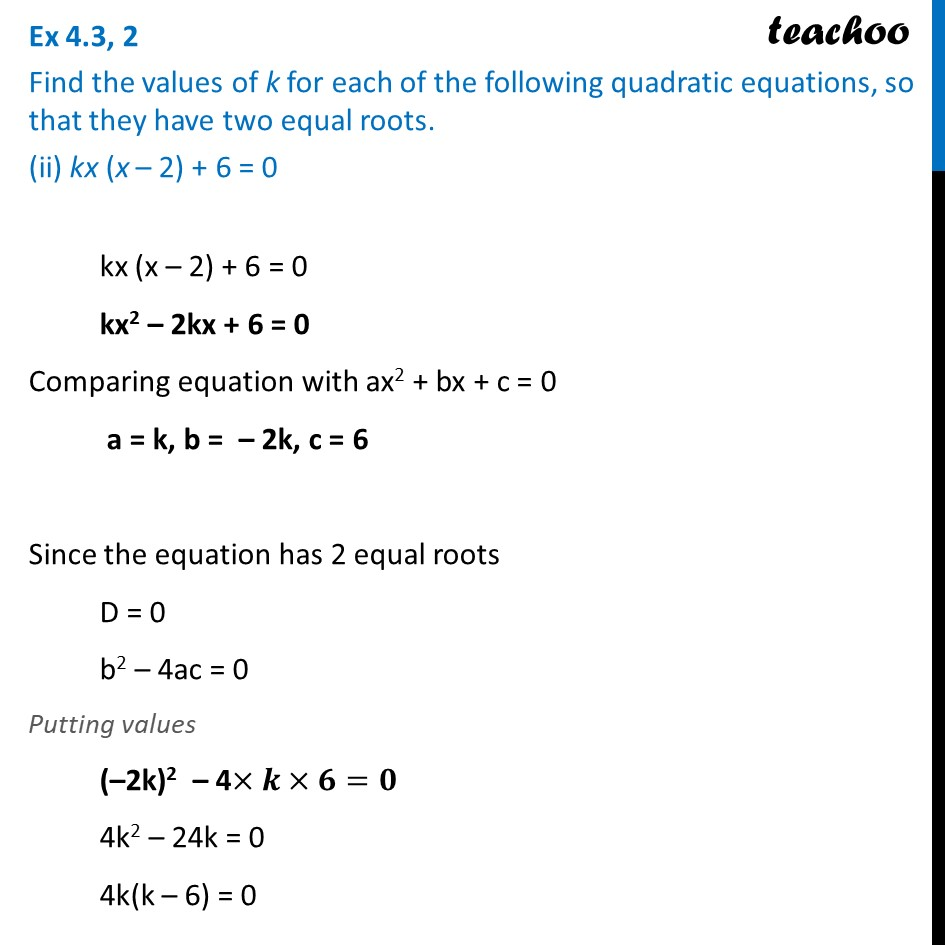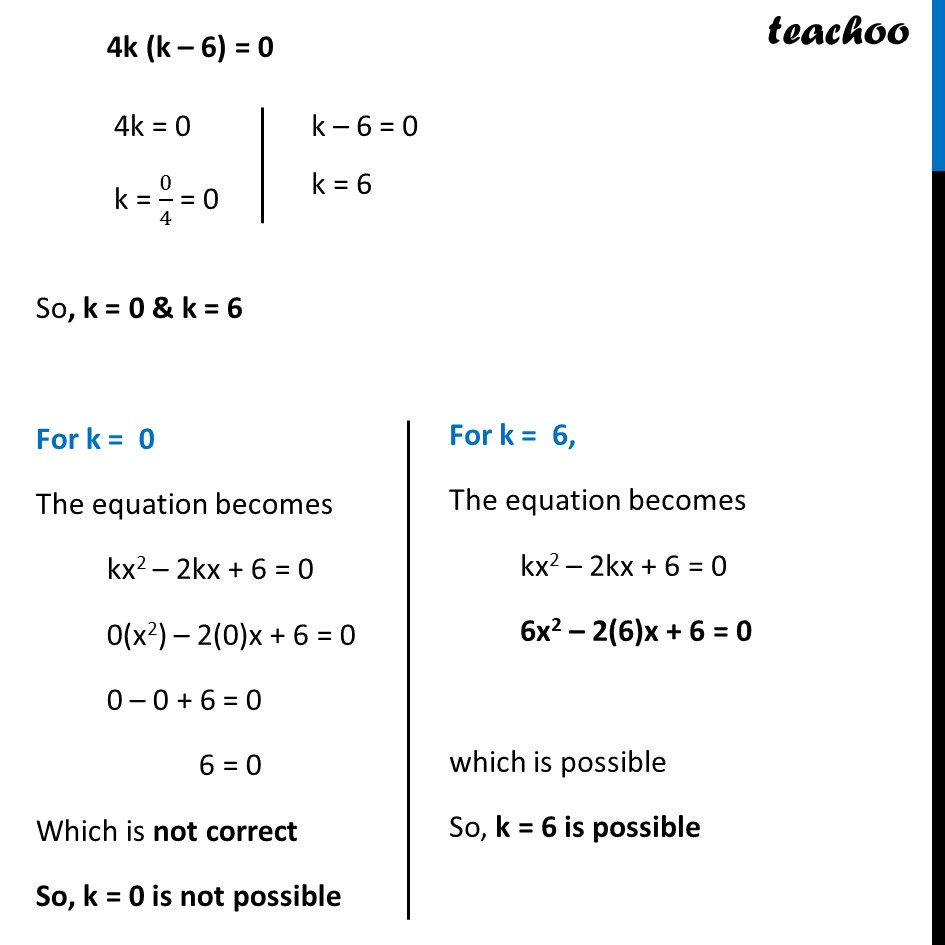Ex 4.3

Chapter 4 Class 10 Quadratic Equations
Serial order wiseLearn in your speed, with individual attention - Teachoo Maths 1-on-1 Class

### Transcript

Ex 4.3, 2 Find the values of k for each of the following quadratic equations, so that they have two equal roots. (ii) kx (x – 2) + 6 = 0 kx (x – 2) + 6 = 0 kx2 – 2kx + 6 = 0 Comparing equation with ax2 + bx + c = 0 a = k, b = – 2k, c = 6 Since the equation has 2 equal roots D = 0 b2 – 4ac = 0 Putting values (–2k)2 – 4×𝒌×𝟔=𝟎 4k2 – 24k = 0 4k(k – 6) = 0 4k (k – 6) = 0 So, k = 0 & k = 6 For k = 0 The equation becomes kx2 – 2kx + 6 = 0 0(x2) – 2(0)x + 6 = 0 0 – 0 + 6 = 0 6 = 0 Which is not correct So, k = 0 is not possible For k = 6, The equation becomes kx2 – 2kx + 6 = 0 6x2 – 2(6)x + 6 = 0 which is possible So, k = 6 is possible